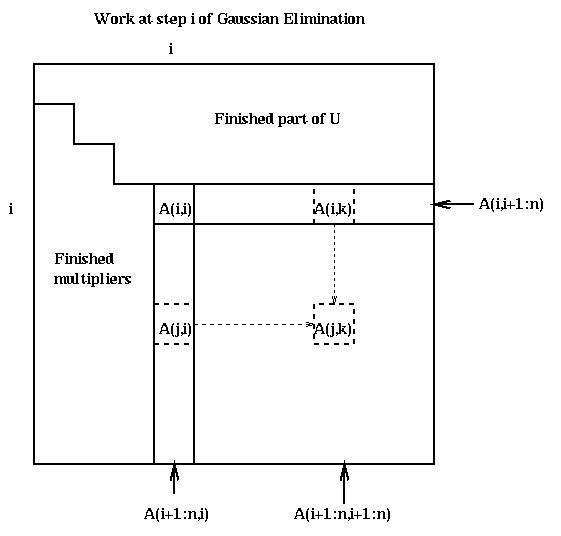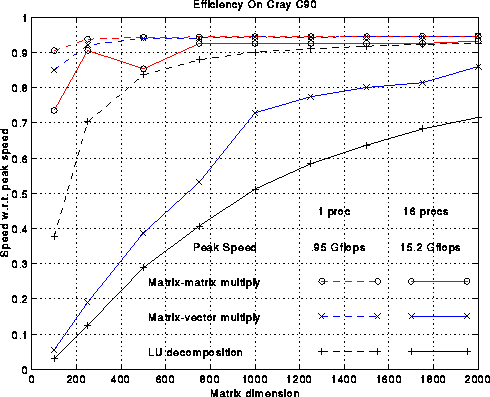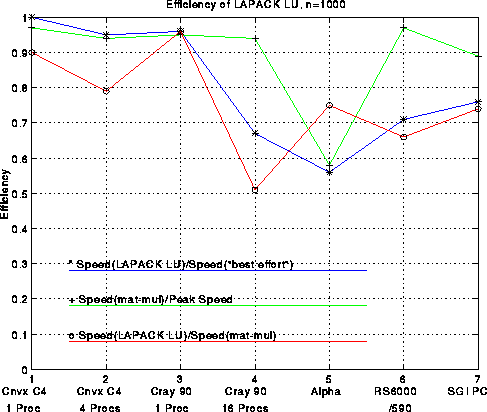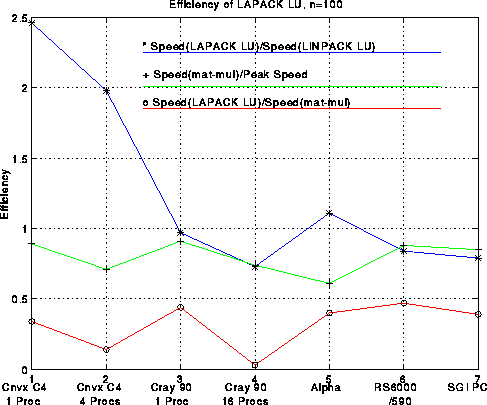# (CS 267, Feb 28 1995)

## Review of last lecture

Recall from last lecture our simplest algorithm for Gaussian elimination, as well as its pictorial representation.
```    for i = 1 to n-1
A(i+1:n, i) = A(i+1:n, i) / A(i,i)
A(i+1:n, i+1:n) = A(i+1:n, i+1:n) - A(i+1:n, i)*A(i, i+1:n)
```This algorithm has several problems, whose solutions we will discuss.

• If A(i,i) is zero, the algorithm cannot proceed. If A(i,i) is tiny, we will also have numerical problems.
• The majority of the work is done by calls to the BLAS2 (rank-one update), which does not exploit a memory hierarchy at well as a BLAS3 operation like matrix-matrix multiplication.
• ## Pivoting to avoid tiny A(i,i)

It is obvious why the algorithm fails when some A(i,i) is exactly zero. Sometimes failure is appropriate: if the entire first column of the matrix is zero, for example, the matrix is singular and the user deserves a warning. On the other hand, if the matrix is perfectly nonsingular, such as
```     A= [ 0 1 ]
[ 1 0 ]
```
then a warning is not deserved.

Even if A(i,i) is not exactly zero, but merely tiny, trouble is possible because of roundoff errors in floating point arithmetic. We illustrate with a 2-by-2 example. Suppose for simplicity we compute in 3-decimal digit arithmetic, so it is easy to see what happens. Let us solve Ax=b where

```     A= [ 1e-4  1 ]  and  b= [ 1 ]  .
[  1    1 ]          [ 2 ]
```
The correct answer to 3 decimal places is easily confirmed to be
```     x = [ 1 ]  .
[ 1 ]
```
The result of LU decomposition on A is as follows, where fl(a op b) is the floating point result of a op b:
```     L = [    1        0 ]  =  [  1   0 ]
[ fl(1/1e-4)  1 ]     [ 1e4  1 ]         ... no error yet
and
U = [ 1e-4       1      ]  =  [ 1e-4    1  ]
[  0    fl(1-1e4*1) ]     [  0    -1e4 ]    ... error in the 4th place
```
The only rounding error committed is replacing 1-1e4*1 by -1e4, an error in the fourth decimal place. Let's see how close L*U is to A:
```     L * U = [  1e-4   1  ]
[   1     0  ]
```
The (2,2) entry is completely wrong. If we continue to try to solve A*x=b, the subsequent solves of L*y=b and U*x=y yield
```     y = [      1      ] = [   1  ]
[ fl(1-1e4*1) ]   [ -1e4 ]    ... error in the 4th place
and
x = [ x1 ] = [ fl((1-x2*1)/1e-4) ] = [ 0 ]    ... no error
[ x2 ]   [  fl(-1e4/(-1e4))  ]   [ 1 ]    ... no error
```
We see that the computed answer is completely wrong, even though there were only two floating errors in the 4th decimal place, and even though the matrix A is "well-conditioned", i.e. changing the entries of A or b by eps<<1 only changes the true solution by O(eps).

The problem can be traced to the first rounding error, U(2,2) = fl(1-1e4*1) = -1e4. Note that if we were to change A(2,2) from 1 to any other number z in the range from -5 to 5, we would get the same value of U(2,2) = fl(z-1e4*1) = -1e4, and so the final computed value of x would be the same, independent of z. In other words, the algorithm "forgets" the value of A(2,2), and so can't possibly get the right answer. This phenomenon is called numerical instability, and must be eliminated to yield a reliable algorithm.

The standard solution to this problem is called partial pivoting, which means reordering the rows of A so that A(i,i) is large at each step of the algorithm. In particular, at the beginning of step i of the algorithm, row i is swapped with row k>i if |A(k,i)| is the largest entry among |A(i:n,i)|. This yields the following algorithm.

```    Gaussian elimination with Partial Pivoting, BLAS2 implementation

for i = 1 to n-1
find and record k where |A(k,i)| = max_{i<=j<=n} |A(j,i)|
if |A(k,i)|=0, exit with a warning that A is singular, or nearly so
if i != k, swap rows i and k of A
A(i+1:n, i) = A(i+1:n, i) / A(i,i)   ...  each quotient lies in [-1,1]
A(i+1:n, i+1:n) = A(i+1:n, i+1:n) - A(i+1:n, i)*A(i, i+1:n)
```

If we apply this algorithm to our 2-by-2 example, we get a very accurate answer.

The LAPACK source for this routine, sgetf2, may be viewed by clicking here.

We may describe this algorithm as computing the factorization P*A=L*U, where L and U are lower and upper triangular as before, and P is a permutation matrix, i.e. the identity matrix with permuted columns. P*A is the matrix A with its rows permuted. As a consequence of pivoting, each entry of L has absolute value at most 1. Using this new factorization of A, solving A*x=b only requires the additional step of permuting b according to P.

Note that we have several choices as to when to swap rows i and k. Indeed, we could use indirect addressing and not swap them at all, but then we couldn't use the BLAS, so this would be slow. Different implementations make different choices about when to swap.

## How to Use BLAS3 in Gaussian Elimination with Partial Pivoting

Most of the work in the algorithm occurs in the rank-one update in its last line, a BLAS2 operation which does 2*m operations on about m data items (where m=(n-i)^2). A standard trick for changing BLAS2 into BLAS3 in linear algebra codes is delayed updating. (In the linear algebra literature, it is also called blocking, but that is a heavily overloaded term in parallel computing!) This means saving up a sequence of BLAS2 operations, and applying them all at once using BLAS3.

For LU decomposition, this means that we will process the matrix in blocks of b columns at a time, rather than one column at a time. b is called the block size. We will do a complete LU decomposition just of the b columns in the current block, essentially using the above BLAS2 code. Then we will update the remainder of the matrix doing b rank-one updates all at once, which turns out to be a single matrix-matrix multiplication, where the common matrix dimension is b. b will be chosen in a machine dependent way to maximize performance. A good value of b will have the following properties:

• b is small enough so that the b columns currently being LU-factorized fit in the fast memory (cache, say) of the machine.
• b is large enough to make matrix-matrix multiplication fast.
• Here is the algorithm.

```    Gaussian elimination with Partial Pivoting, BLAS3 implementation

for ib = 1 to n-1 step b     ... process matrix b columns at a time
end = min(ib+b-1,n)      ... points to end of current block of b columns

for i = ib to end        ... LU factorize A(i:n,i:end) using BLAS2
find and record k where |A(k,i)| = max_{i<=j<=n} |A(j,i)|
if |A(k,i)|=0, exit with a warning that A is singular, or nearly so
if i != k, swap rows i and k of A
A(i+1:n, i) = A(i+1:n, i) / A(i,i)
A(i+1:n, i+1:end) = A(i+1:n, i+1:end) - A(i+1:n, i)*A(i, i+1:end)
... only update columns i+1 to end

Let LL refer to the b-by-b lower triangular matrix whose subdiagonal
entries are stored in A(ib:end,ib:end), and with 1s on the diagonal
A(ib:end, end+1:n) = LL \ A(ib:end, end+1:n)
... perform delayed update of A(ib_end, end+1:n) by
... solving n-end triangular systems of equations (Matlab notation)

A(end+1:n, end+1:n) = A(end+1:n, end+1:n) - A(end+1:n,ib:end)*A(ib(end,end+1:n)
... perform delayed update of rest of matrix using
... matrix-matrix multiplication
```

The LU factorization of A(i:n,i:end) uses the same algorithm as before. Solving n-end triangular systems of equations is a single call to a BLAS3 subroutine designed for that purpose. No work or data motion is required to refer to LL; it is done with a pointer. When n>>b, almost all the work is done in the final line, which multiplies an (n-end)-by-b matrix times a b-by-(n-end) matrix. Here is a picture, where the final line subtracts the product of the blue and pink submatrices from the green submatrix.The LAPACK source for this routine, sgetrf, may be viewed by clicking here.

## Performance of LAPACK LU Decomposition

We discuss the performance of the LAPACK LU decomposition routine, as well as some other routines. The first plot below shows the performance of matrix-matrix multiplication, matrix-vector multiplication, and LU decomposition on the Cray C90, both on 1 processor (dotted lines) and on 16 processors (solid lines). The speed of each routine divided by peak machine speed (efficiency) is shown. Matrix-matrix multiply is fastest, running at over 90% of peak for large matrices, followed by matrix-vector multiplication, and finally LU decomposition. The most important feature of this plot is that efficiency increases with increasing dimension. This is a common phenomenon for matrix-matrix multiply based algorithms as we discussed in Lecture 2. The second important feature is that efficiency is higher on one processor than 16 processors. This happens because keeping the matrix dimension fixed and increasing the number of processors means there is less work to do per processor.Now we fix the dimension at N=1000 and compare the efficiencies on a variety of architectures, from large vector processors like the Cray C90 and Convex C4640, to shared-memory multiple processor versions of both machines, to workstations like the DEC Alpha, IBM RS6000/590, and SGI Power Challenge. The vital statistics of these machines are given in the following table. All results are for 64-bit precision.

```    Machine            #Procs  Clock     Peak    Block
Speed   Mflops   Size b
(MHz)
----------------------------------------------------
Convex C4640          1      135      810       64
Convex C4640          4      135     3240       64
Cray C90              1      240      952      128
Cray C90             16      240    15238      128
DEC Alpha 3000-500X   1      200      200       32
IBM RS 6000/590       1       66      264       64
SGI Power Challenge   1       75      300       64
```
The figure below plots several efficiency measures for the machines in the table. There is one column in the plot for each machine. First consider the green line, which measures the fraction of peak performance attained by 1000-by-1000 matrix-multiplication. We use the best matrix-multiplication available for the machine, typically supplied by the vendor. For all machines except the DEC Alpha 3000-500X, efficiency exceeds 90%.Now consider the red line, which is the speed of LU decomposition divided by the speed of matrix-multiply. The block size b is given in the above table; it was chosen by brute force search to optimize the performance. This curve measures how well we achieved our goal of making LU decomposition as fast as the matrix-multiply in its inner loop. The best efficiency, 95%, is attained on a single processor Cray C90, and the worst efficiency, 50%, is attained on a 16 processor Cray C90. This drop in performance is due to the fact that multiplying (n-i)-by-128 times 128-by-(n-i) matrices does not yield enough work to keep all 16 processors busy, although it is enough for 1 processor. The workstations (Alpha, RS6000, SGI) are all close to 70% efficient, which is reasonably satisfactory.

Finally, the blue line measures the efficiency of our LU decomposition with respect to "best effort", which means a version of LU decomposition crafted by each vendor especially for its machine. These vendor-supplied codes use very similar techniques to the ones described above, but try to improve performance by using a variable block size, assembly language, or an algorithm corresponding to a different permutation of the 3 nested loops in the original algorithm (all 3!=6 permutations lead to viable algorithms). The blue curve measures the extent to which we have sacrificed performance to gain portability: We have not lost much on the large vector machines, but 25%-30% on the workstations. The vendors typically invest a large amount of effort in optimizing 1000-by-1000 LU because it is one of the most popular benchmarks used to compare high performance machines: the LINPACK Benchmark. It would be nice if vendors had the resources to highly optimize all the library routines one might want to use, but few do, so portable high-performance libraries remain of great interest.

The LINPACK Benchmark is named after the predecessor of LAPACK. It originally consisted just of timings for 100-by-100 matrices, solved using a fixed Fortran program (sgefa) from the LINPACK linear algebra library. The data is an interesting historical record, with literally every machine for the last 2 decades listed in decreasing order of speed, from the largest supercomputers to a hand-held calculator. As machines grew faster and vendors continued to optimize their LINPACK benchmark performance (sometimes by using special compiler flags that worked only for the LINPACK benchmark!), the timings for 100-by-100 matrices grew less and less indicative of typical machine performance. Then 1000-by-1000 matrices were introduced. In contrast to the 100-by-100 case, which required vendors to use identical Fortran code, vendors were permitted to modify Gaussian elimination anyway they wanted for the 1000-by-1000 case, provided they got an accurate enough answer. Finally, a third benchmark was added for large parallel machines, which measured their speed on the largest linear system that would fit in memory, as well as the size of the system required to get half the Mflop rate of the largest matrix.

Here is a plot analogous to the last one but for 100-by-100 matrices. Generally speaking, efficiencies are lower for n=100 than n=1000, dramatically so for large machines like the Cray, less so for workstations. Rather than comparing LAPACK performance to the ``best effort'', it is compared to the LINPACK benchmark discussed above, i.e. performance using the LINPACK benchmark code sgefa. This ratio is as high as 2.46 for, but is as low as .73, a testimony to the efforts of the manufacturers to tune their compilers to work well on sgefa.For an analysis and summary of the performance of other LAPACK routines besides LU, click here.